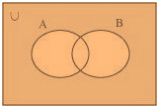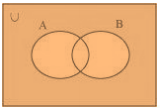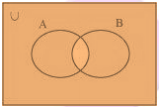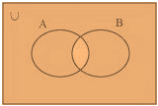# NCERT Solutions For Class 11 Math Chapter – 1 Exercise – 1.5

#### NCERT Solutions For Class 11 Math Chapter – 1 Exercise – 1.5

1. Let U = {1, 2, 3; 4, 5, 6, 7, 8, 9}, A = {1, 2, 3, 4}, B = {2, 4, 6, 8} and C = {3, 4, 5, 6}. Find

(i) A’

(ii) B’

(iii) (A U C)’

(iv) (A U B)’

(v) (A’)’

(vi) (B – C)’

Solution:

It is given that

U = {1, 2, 3, 4, 5, 6, 7, 8, 9}

A = {1, 2, 3, 4}

B = {2, 4, 6, 8}

C = {3, 4, 5, 6}

(i) A’ = {5, 6, 7, 8, 9}

(ii) B’ = {1, 3, 5, 7, 9}

(iii)

A U C = {1, 2, 3, 4, 5, 6}

So we get

(A U C)’ = {7, 8, 9}

(iv) A U B = {1, 2, 3, 4, 6, 8}

So we get

(A U B)’ = {5, 7, 9}

(v) (A’)’ = A = {1, 2, 3, 4}

(vi) B – C = {2, 8}

So we get

(B – C)’ = {1, 3, 4, 5, 6, 7, 9}

2. If U = {a, b, c, d, e, f, g, h}, find the complements of the following sets:

(i) A = {a, b, c}

(ii) B = {d, e, f, g}

(iii) C = {a, c, e, g}

(iv) D = {fgha}
Solution:

(i) A = {a, b, c}

So we get

A’ = {d, e, f, g, h}

(ii) B = {d, e, f, g}

So we get

B’ = {a, b, c, h}

(iii) C = {a, c, e, g}

So we get

C’ = {b, d, f, h}

(iv) D = {fgha}

So we get

D’ = {b, c, d, e}

3. Taking the set of natural numbers as the universal set, write down the complements of the following sets:

(i) {xx is an even natural number}

(ii) {xx is an odd natural number}

(iii) {xx is a positive multiple of 3}

(iv) {xx is a prime number}

(v) {xx is a natural number divisible by 3 and 5}

(vi) {xx is a perfect square}

(vii) {xx is perfect cube}

(viii) {xx + 5 = 8}

(ix) {x: 2x + 5 = 9}

(x) {xx ≥ 7}

(xi) {xx ∈ N and 2x + 1 > 10}

Solution:

We know that

U = N: Set of natural numbers

(i) {xx is an even natural number}´ = {xx is an odd natural number}

(ii) {xx is an odd natural number}´ = {xx is an even natural number}

(iii) {xx is a positive multiple of 3}´ = {xx ∈ N and x is not a multiple of 3}

(iv) {xx is a prime number}´ ={xx is a positive composite number and x = 1}

(v) {xx is a natural number divisible by 3 and 5}´ = {xx is a natural number that is not divisible by 3 or 5}

(vi) {xx is a perfect square}´ = {xx ∈ N and is not a perfect square}

(vii) {xx is a perfect cube}´ = {xx ∈ N and is not a perfect cube}

(viii) {xx + 5 = 8}´ = {xx ∈ N and x ≠ 3}

(ix) {x: 2x + 5 = 9}´ = {xx ∈ N and x ≠ 2}

(x) {xx ≥ 7}´ = {xx ∈ N and x < 7}

(xi) {xx ∈ N and 2x + 1 > 10}´ = {xx ∈ N and ≤ 9/2}

4. If U = {1, 2, 3, 4, 5,6,7,8, 9}, A = {2, 4, 6, 8} and B = {2, 3, 5, 7}. Verify that

(i) (A U B)’ = A’ ∩ B’

(ii) (A ∩ B)’ = A’ U B’

Solution:

It is given that

U = {1, 2, 3, 4, 5,6,7,8, 9}

A = {2, 4, 6, 8}

B = {2, 3, 5, 7}

(i) (A U B)’ = {2, 3, 4, 5, 6, 7, 8}’ = {1, 9}

A’ ∩ B’ = {1, 3, 5, 7, 9} ∩ {1, 4, 6, 8, 9} = {1, 9}

Therefore, (A U B)’ = A’ ∩ B’.

(ii) (A ∩ B)’ = {2}’ = {1, 3, 4, 5, 6, 7, 8, 9}

A’ U B’ = {1, 3, 5, 7, 9} U {1, 4, 6, 8, 9} = {1, 3, 4, 5, 6, 7, 8, 9}

Therefore, (A ∩ B)’ = A’ U B’.

5. Draw appropriate Venn diagram for each of the following:

(i) (A U B)’

(ii) A’ ∩ B’

(iii) (A ∩ B)’

(iv) A’ U B’

Solution:

(i) (A U B)’(ii) A’ ∩ B’(iii) (A ∩ B)’(iv) A’ U B’6. Let U be the set of all triangles in a plane. If A is the set of all triangles with at least one angle different from 60°, what is A’?

Solution:

A’ is the set of all equilateral triangles.

7. Fill in the blanks to make each of the following a true statement:

(i) A U A’ = ……..

(ii) Φ′ ∩ A = …….

(iii) A ∩ A’ = …….

(iv) U’ ∩ A = …….

Solution:

(i) A U A’ = U

(ii) Φ′ ∩ A = U ∩ A = A

So we get

Φ′ ∩ A = A

(iii) A ∩ A’ = Φ

(iv) U’ ∩ A = Φ ∩ A = Φ

So we get

U’ ∩ A = Φ